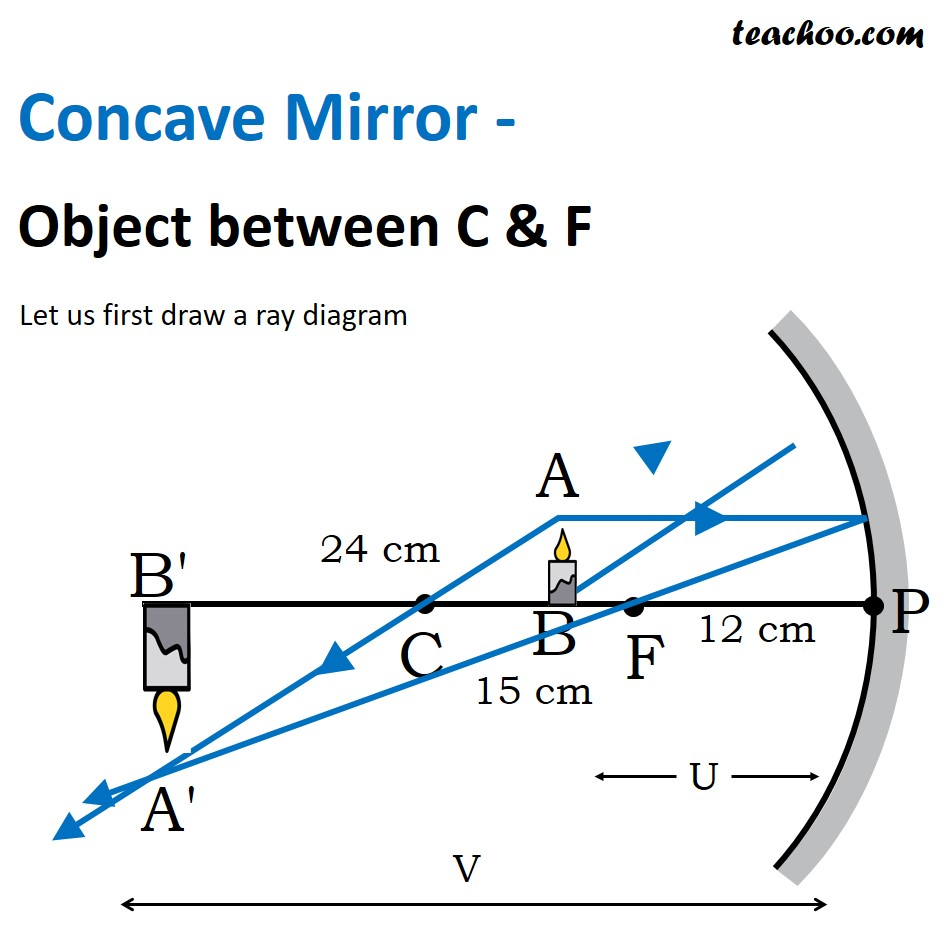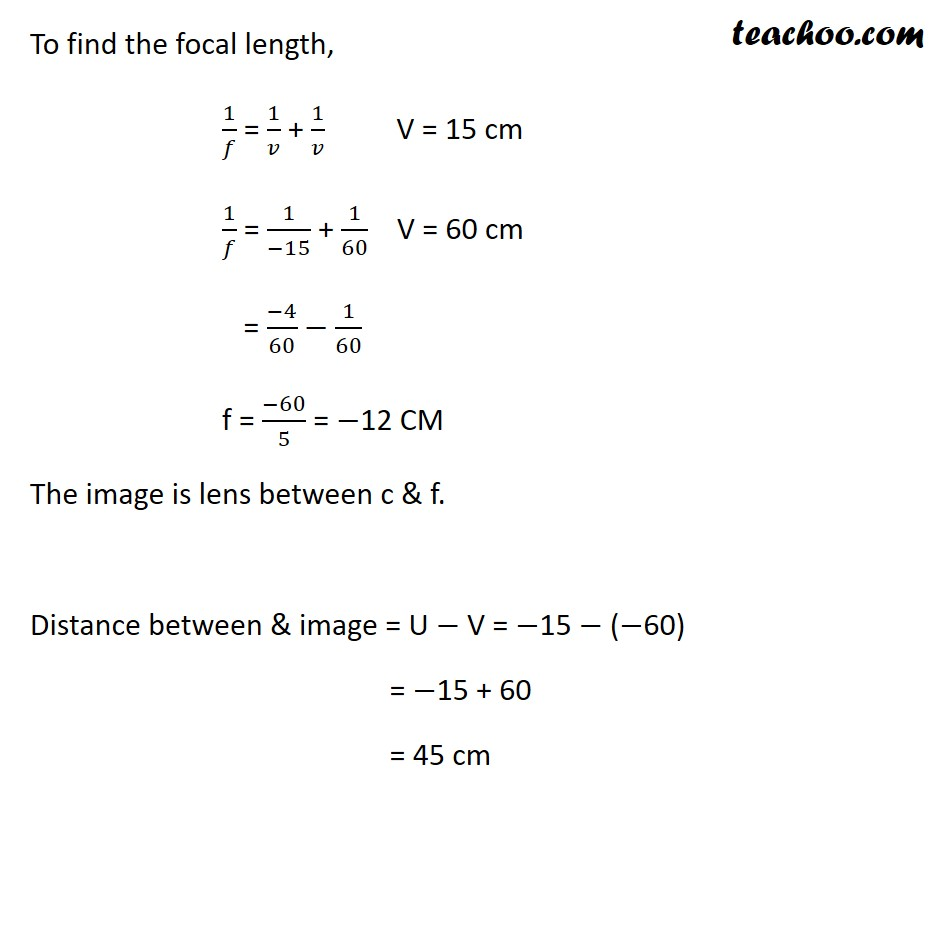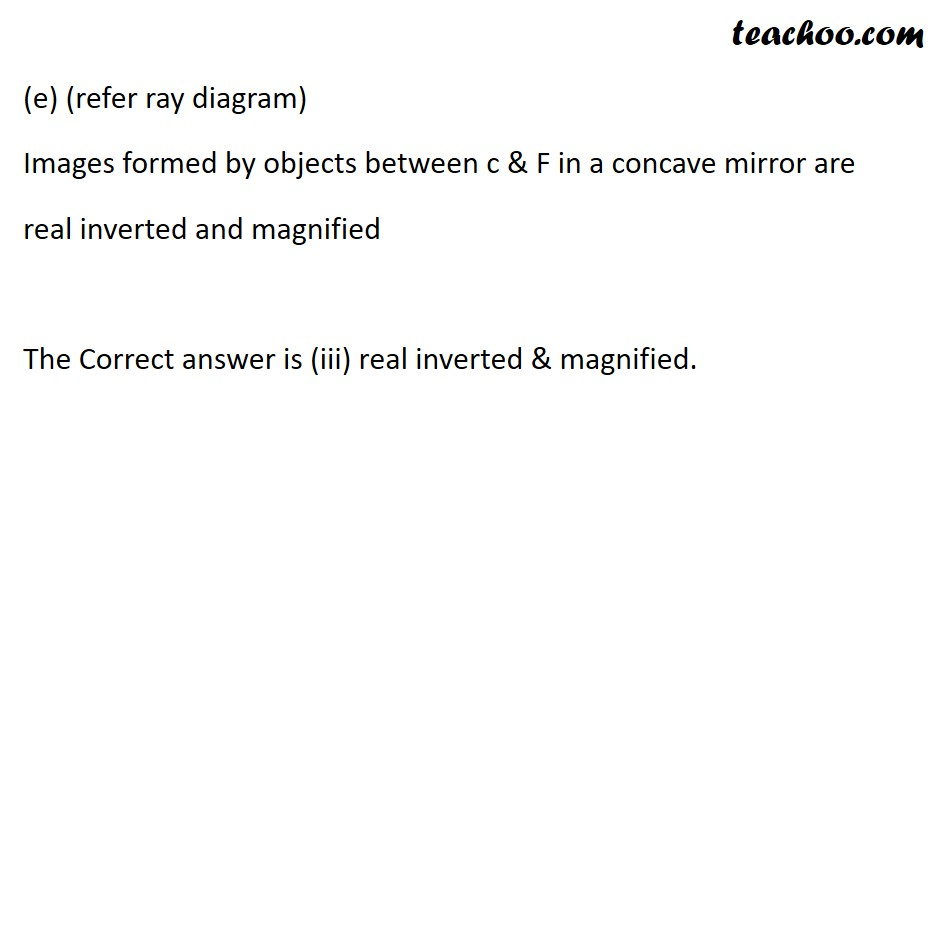Case Based Questions (MCQ)

Class 10
Chapter 10 Class 10 - Light - Reflection and Refraction

## A student wants to project the image of a candle  flame on a screen 60 cm in front of a mirror by  keeping the flame at a distance of 15 cm from its  pole.

### (iv) none of the above

Ans.

Since the student wants image on a screen , it has to be a real image

Now, let’s check both mirrors

• Convex mirrors always produce virtual images . It cannot be used to produce images on the screen.
• Concave mirrors produce real images on the screen in all cases except when the object is between pole and focus , therefore students should use a concave mirror because it forms real images.
• Plane mirrors always form virtual images. So, it cannot be used

So, the correct answer is (iii) Concave mirror

### (iv) + 900

Ans.

So, the correct answer is (i) – 4

### (iv) virtual, real

Ans.

In a concave mirror

• When object is kept between Focus and Pole , image formed is virtual
• When object is kept beyond focus (between C and F, at C and beyond C), image formed is real

So, the correct answer is (iv) virtual, real

### (d) Draw  a ray diagram for the given scenario.### (iv) 0 cm

Ans.Given

Object distance, u = -15cm

Image distance, v = -60cm

Now,

Distance between object and image = u - v

= -15 - (-60)

= 45cm

The correct answer is (ii) 15 cm

### (iv) real, erect and diminished

Ans.

• Since the image is formed on a screen , it is real .

Also, we calculated the magnification of the image as m= -4.

• Since M = image size/object size, it is clear that the image is 4 times larger than the object.
• Hence it is magnified.
• The negative sign in the magnification means that the image is inverted.

Thus, the correct answer is (i) real, inverted and magnified

Learn in your speed, with individual attention - Teachoo Maths 1-on-1 Class